# 2 6 Notes Special Functions A function that

• Slides: 13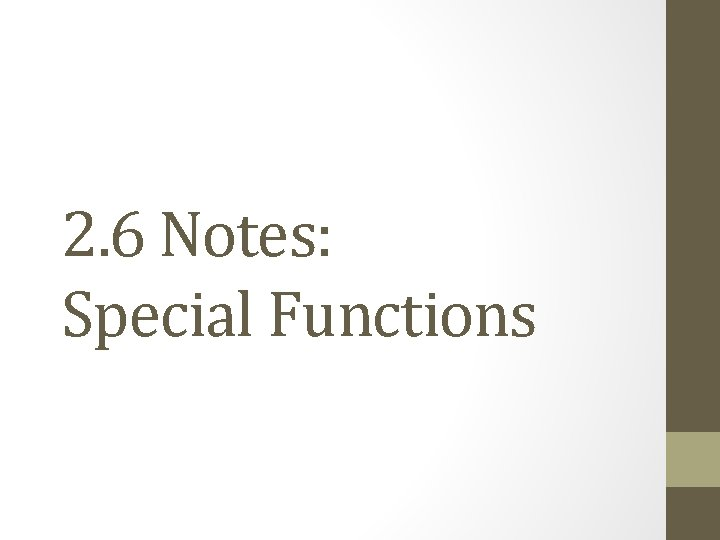2. 6 Notes: Special Functions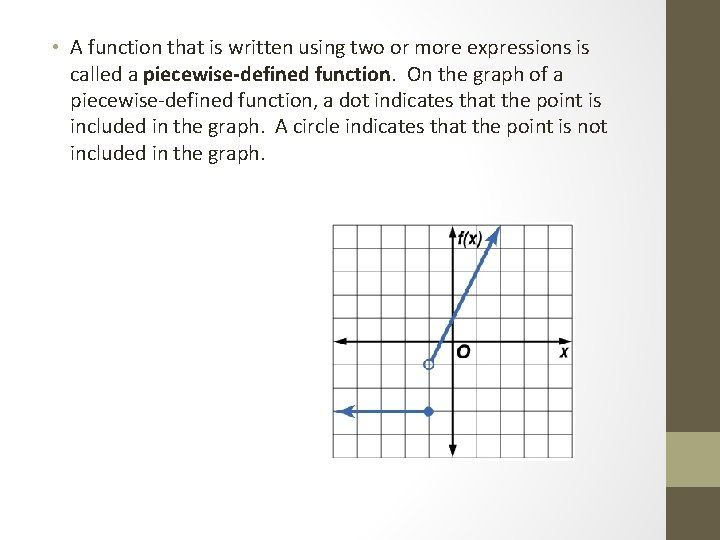• A function that is written using two or more expressions is called a piecewise-defined function. On the graph of a piecewise-defined function, a dot indicates that the point is included in the graph. A circle indicates that the point is not included in the graph.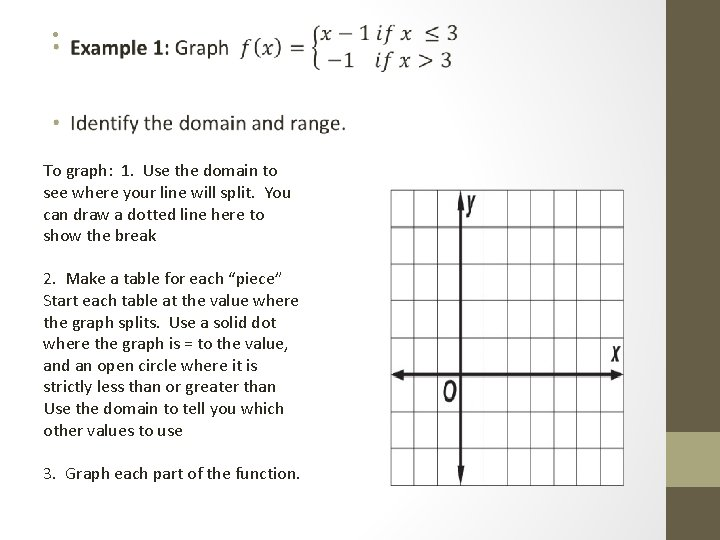• To graph: 1. Use the domain to see where your line will split. You can draw a dotted line here to show the break 2. Make a table for each “piece” Start each table at the value where the graph splits. Use a solid dot where the graph is = to the value, and an open circle where it is strictly less than or greater than Use the domain to tell you which other values to use 3. Graph each part of the function.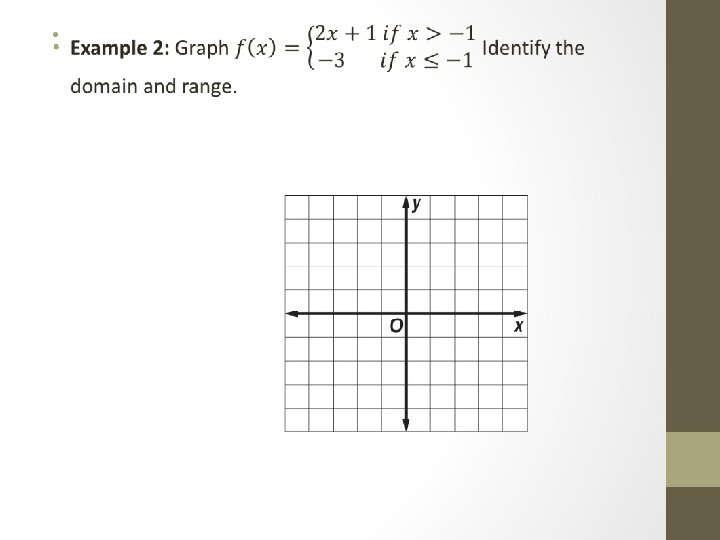• A piecewise-linear function only contains a single expression. A common piecewise-linear function is the step function. The graph of a step function consists of line segments.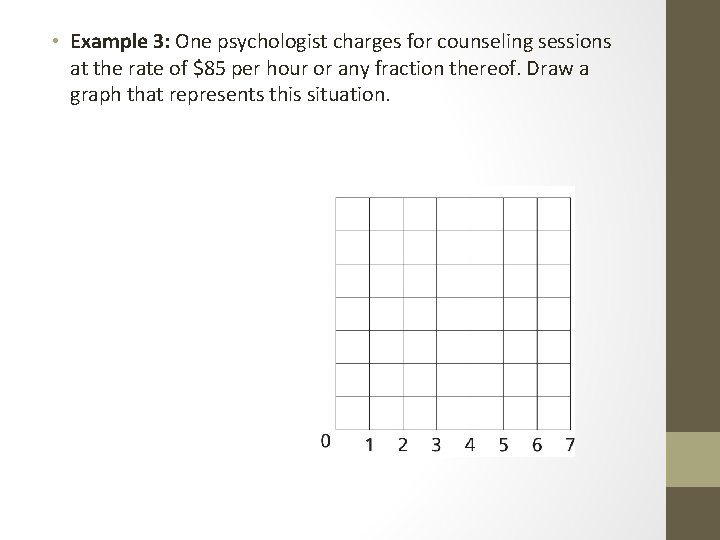• Example 3: One psychologist charges for counseling sessions at the rate of \$85 per hour or any fraction thereof. Draw a graph that represents this situation.• Another piecewise-linear function is the absolute value function. An absolute value function is a function that contains an algebraic expression within absolute value symbols.• Example 5: Graph y = |x| + 1. Identify the domain and range.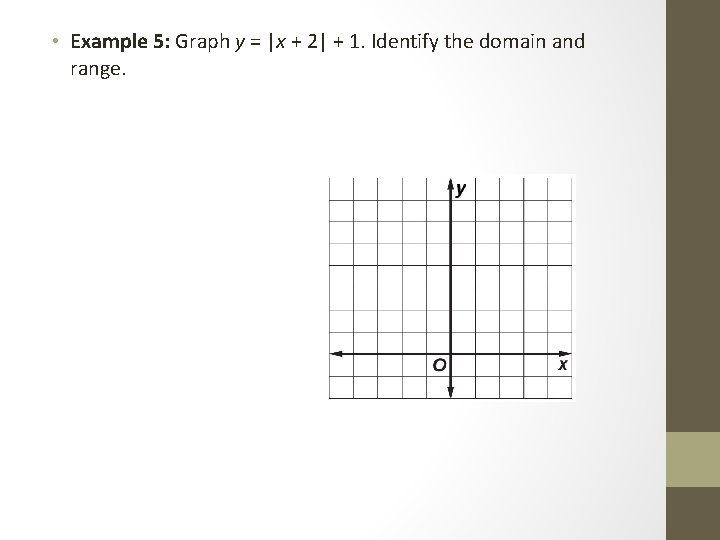• Example 5: Graph y = |x + 2| + 1. Identify the domain and range.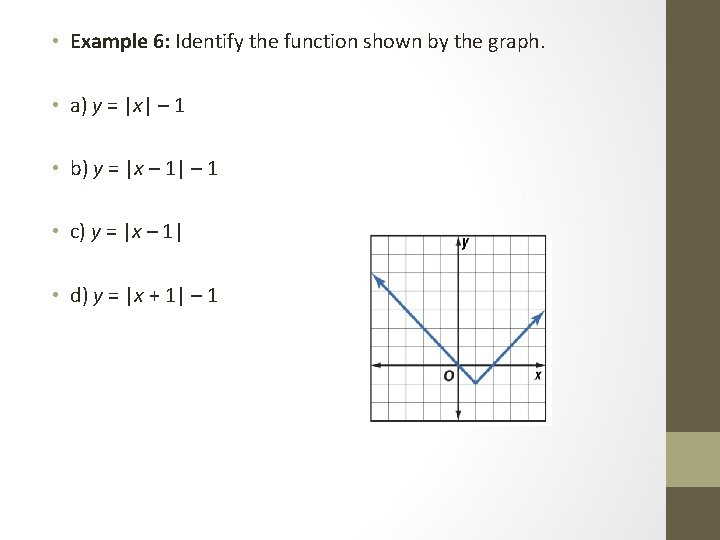• Example 6: Identify the function shown by the graph. • a) y = |x| – 1 • b) y = |x – 1| – 1 • c) y = |x – 1| • d) y = |x + 1| – 1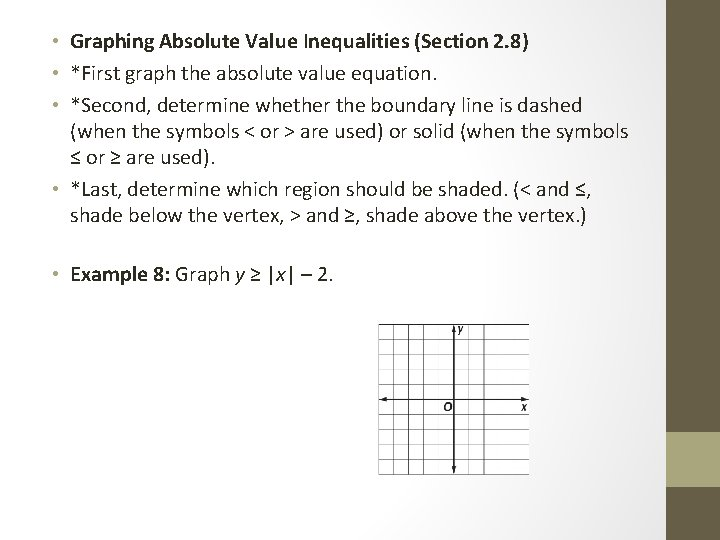• Graphing Absolute Value Inequalities (Section 2. 8) • *First graph the absolute value equation. • *Second, determine whether the boundary line is dashed (when the symbols < or > are used) or solid (when the symbols ≤ or ≥ are used). • *Last, determine which region should be shaded. (< and ≤, shade below the vertex, > and ≥, shade above the vertex. ) • Example 8: Graph y ≥ |x| – 2.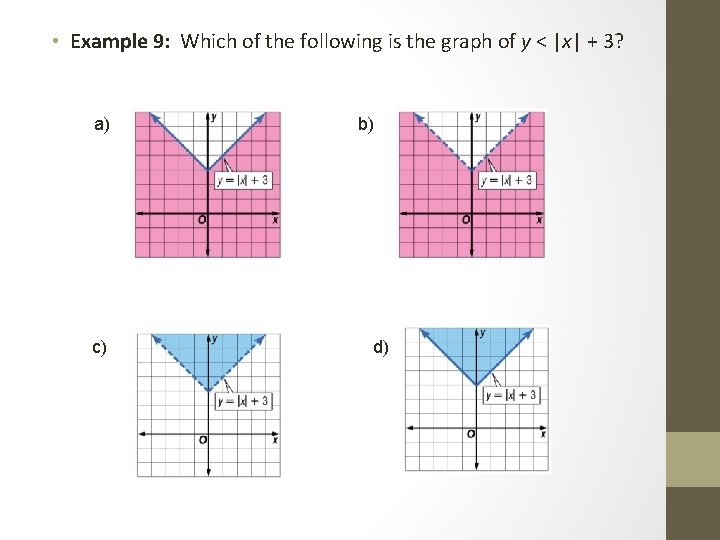• Example 9: Which of the following is the graph of y < |x| + 3? a) c) b) d)